# 深度学习的数学 (4)：神经网络的思想 1-4(什么是神经网络)

《深度学习的数学》基于丰富的图示和具体示例，通俗易懂地介绍了深度学习相关的数学知识。第 1 章介绍神经网络的概况；第 2 章介绍理解神经网络所需的数学基础知识；第 3 章介绍神经网络的 * 优化；第 4 章介绍神经网络和误差反向传播法；第 5 章介绍深度学习和卷积神经网络。书中使用 Excel 进行理论验证，帮助读者直观地体验深度学习的原理。

### 神经网络

• 将神经单元的多个输入 $x_1,x_2,\cdots,x_n$ 整理为加权输入 $z$
$z=w_1x_1+w_2x_2+\cdots+w_nx_n+b\quad\quad\quad\quad\quad\quad\quad\quad(1)$
其中 $w_1,w_2,\cdots,x_n$ 为权重，$b$ 为偏置，$n$ 为输入的个数。
• 神经单元通过激活函数 $a(z)$，根据加权输入 $z$ 输出 $y$
$y=a(z)\quad\quad\quad\quad\quad\quad\quad\quad(2)$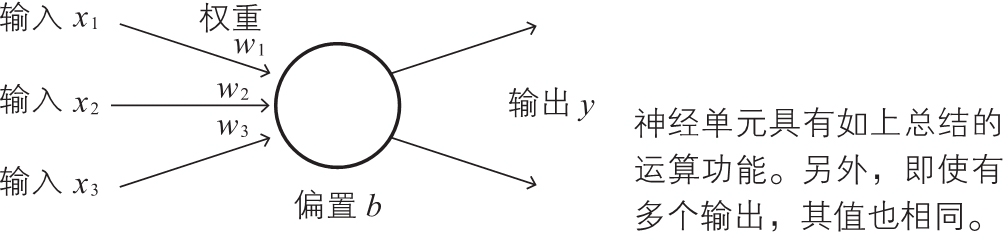### 神经网络各层的职责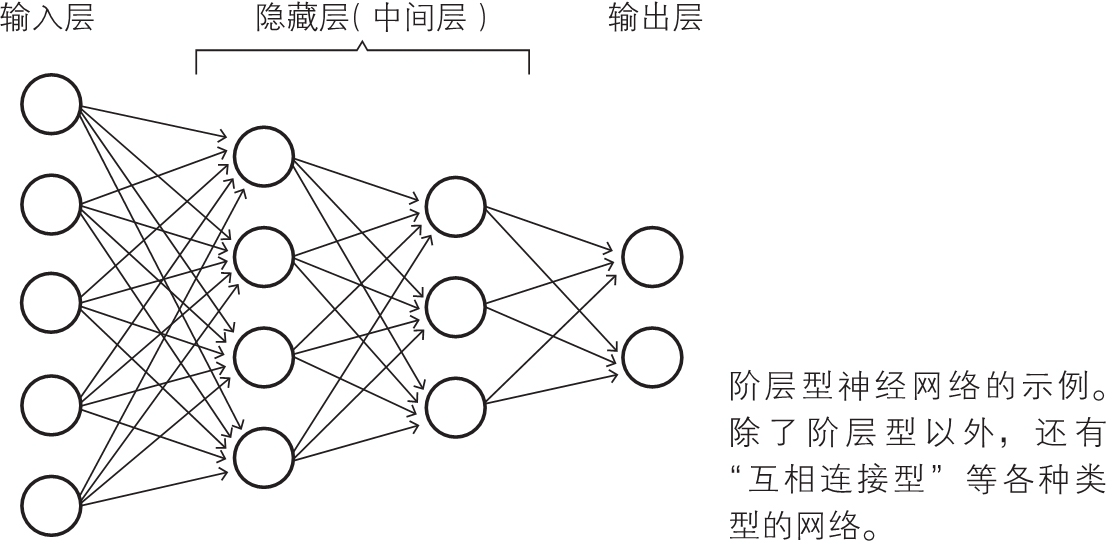### 考察具体的例子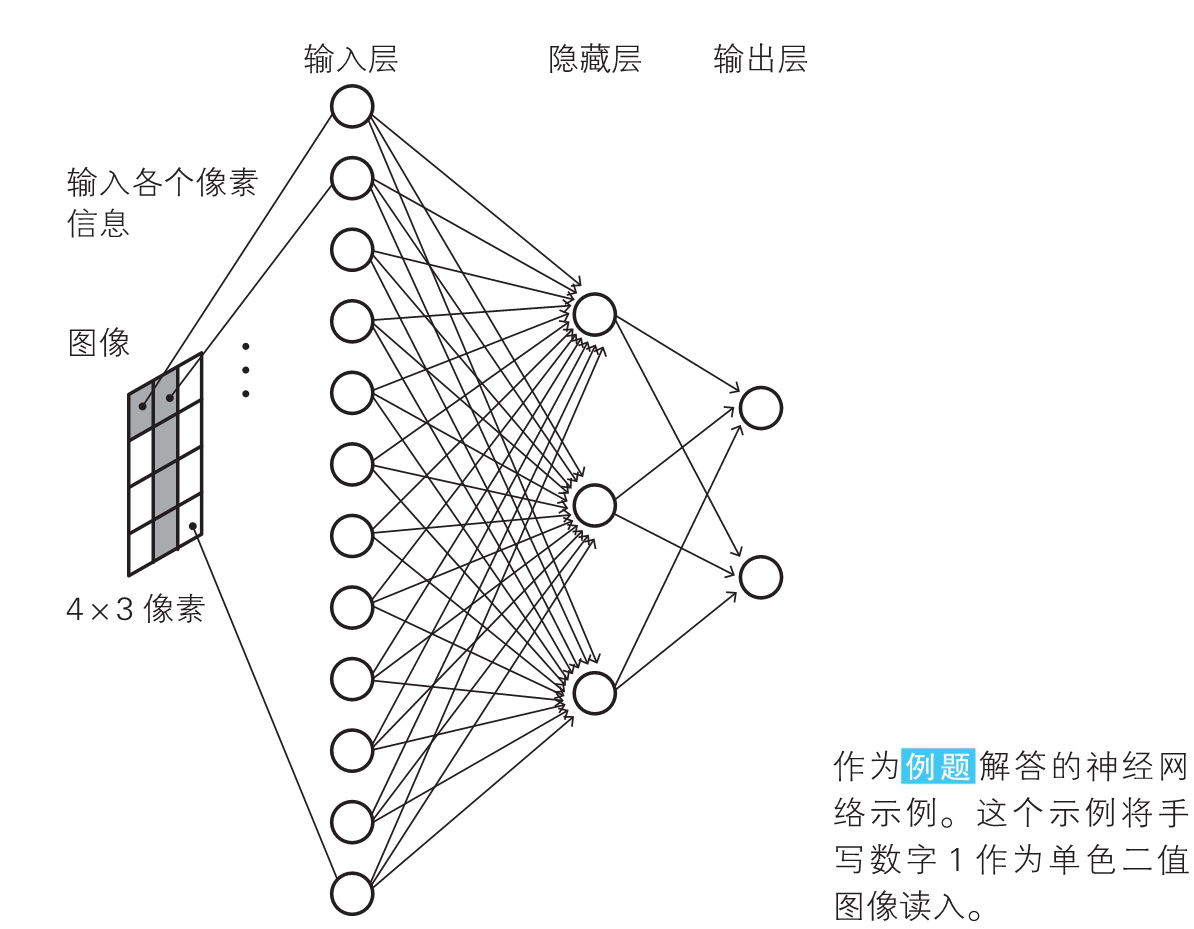### 解答示例中输入层的含义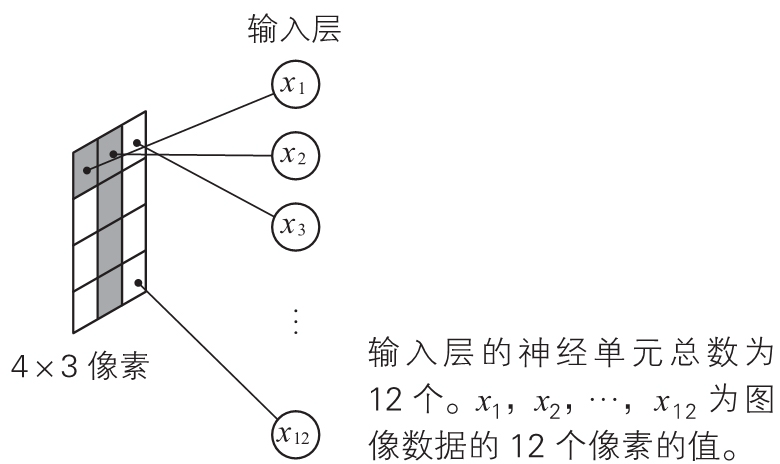### 解答示例中输出层的含义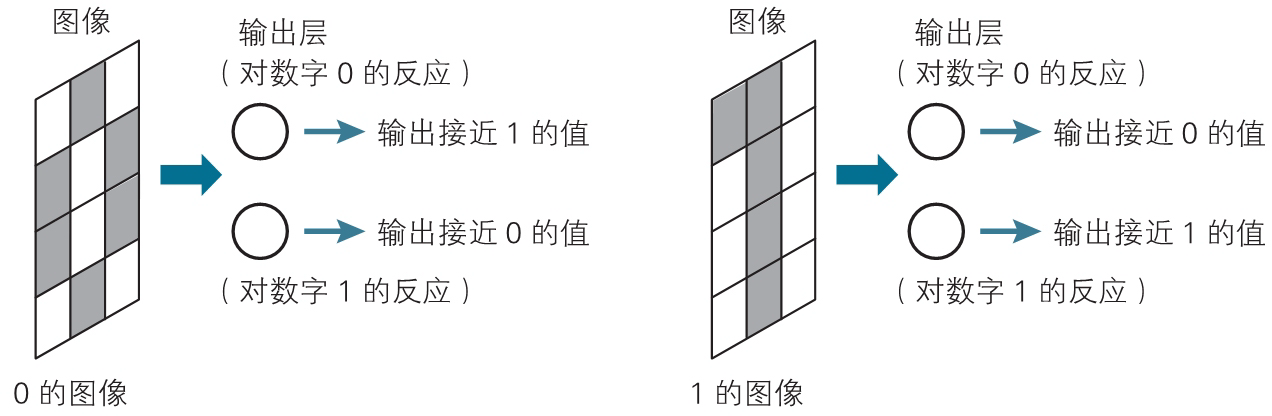### 解答示例中隐藏层的含义